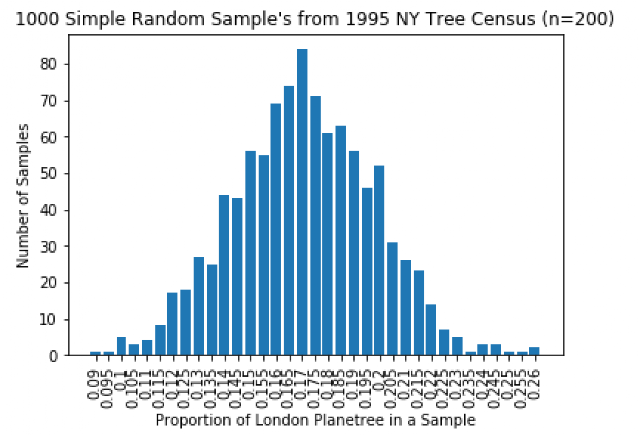### Probability Distribution Example [w/ the NYC Tree Census]

Probability distributions take samples from a population, calculating the proportions of a certain item within each sample in order to estimate the parameter proportion. The parameter proportion is defined to be the actual proportion within the sample. The sample size of the amount of samples you take from the population for each collection of items (for your estimate) has a significant impact on your ability to predict the proportion. This can be demonstrated with a simple computer simulation.London Planetree. Source

According to the 2015 NYC Tree Census, the most popular tree is the London planetree with 87014 member out of a total of 683788 total individual trees. This means our true parameter is 12.7%. In order to better estimate the figure from our proportion distribution, we will need to essentially make sure our probability distribution is normal.

This is accomplished via the normality test which states that: n*p >= 10 && n*(1-p) >= 10

where n is the sample size and p is the true proportion (parameter). If you aren't sure what p is, you will eventually reach a normal curve by increasing the sample size and re-running your simulation. You will also want to make sure that you are drawing samples from the same population each time you draw a sample (maintaining independence).

This principle is at work in the following example with the London planetrees:Proportion of the London Planetree in the 2015 NYC Tree Census (n=5)

Since 5*.127 is not greater than ten, this simulation produces a graph that lacks normality.Proportion of London Planetree in the 2015 NYC Tree Census (n=20)

Although 20*.127 is still not greater than ten, this simulation produces a graph that is closer to normal and less skewed than the previous example. This indicates that we are closer to achieving to our goal as we increase sample size.Proportion of London Planetree in the 2015 NYC Tree Census (n=200)

Since 200*.127>10, the condition for normality has been reached and we can form better estimates for the actual parameter proportion.

Bonus Graphs (Sample Proportions for the 1995 and 2005 Census):Proportion of London Planetree in the 2005 NYC Tree Census (n=200)Proportion of London Planetree in the 1995 NYC Tree Census (n=200)
Feel free to remix the code with the three different datasets to try out these simulations for yourself (i.e. utilizing simulations for probability distributions).

Program Code

import pandas as pd
import matplotlib.pyplot as plt
from collections import Counter
import numpy as np
from numpy.random import choice

def getKey(item):
return item

def main():
treeSpecies = list(treeStuff["spc_common"])
treeSpeciesCombined = Counter(treeSpecies)
j = sorted(treeSpeciesCombined.items(),key=getKey)
treeSpecies2 = []
treeCount = []
for i in j:
i = list(i)
if (isinstance(i,float)):
i = "Unknown"
treeSpecies2.append(i)
treeCount.append(i)
counts = []
for k in range(0,1000):
draw = choice(treeSpecies2, 200, p = [x / float(sum(treeCount)) for x in treeCount]) # where you set sample size
count = sum(np.char.count(draw,sub="London planetree"))
counts.append(count/float(len(draw)))
probabilities = Counter(counts)
l = sorted(probabilities.items())
probCategories = []
probValues = []
for r in l:
r = list(r)
probCategories.append(r)
probValues.append(r)
x_pos = [e for e, _ in enumerate(probCategories)]
plt.xticks(x_pos, probCategories,rotation='vertical')
plt.xlabel("Proportion of London Planetree in a Sample")
plt.ylabel("Number of Samples")
plt.title("1000 Simple Random Sample's from 2015 NY Tree Census (n=200)")
plt.bar(x_pos,probValues)
plt.show()

main()


Data Source: See this file on Kaggle for the NYC Tree Census Data.